Function Repository Resource:

# LinearConstraints

Determine the consistency equations required for a system of linear equations to have a solution

Contributed by: Dennis M Schneider
 ResourceFunction["LinearConstraints"][mat,vec] determines the constraint equations that a vector vec must satisfy for the matrix equation mat.x==vec to have a solution. ResourceFunction["LinearConstraints"][mat,b] returns ResourceFunction["LinearConstraints"][mat,{b1,b2,…}] or ResourceFunction["LinearConstraints"][mat,{b,b,…}] if the option Subscript is set to False.

## Examples

### Basic Examples (3)

Find the constraint equations that the vector {a,b,c} must satisfy to be in the column space of the matrix:

 In:=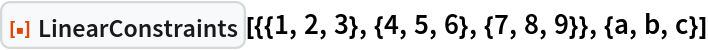Out=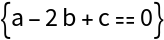When the second argument is a symbol, the second argument is replaced by a subscripted vector in that symbol:

 In:=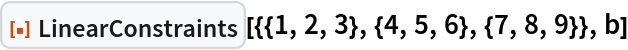Out=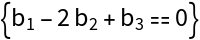When SubscriptFalse, the second argument is replaced by the array vector {b,b,b}:

 In:=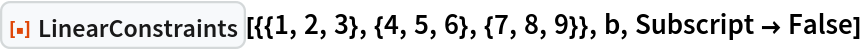Out=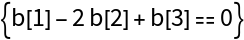Setting the option PrintDisplayTrue prints the matrix form of the reduced matrix that produced the constraint equations:

 In:=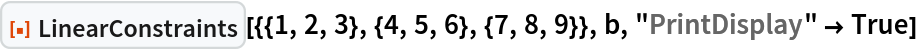Out=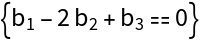The print statement generates a matrix displayed in MatrixForm. To convert it to a matrix, paste a copy of the matrix into a new cell and select Cell ConvertTo StandardForm (Shift + Ctrl + N):

 In:=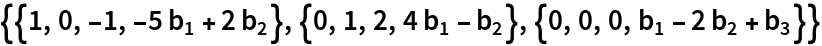Out=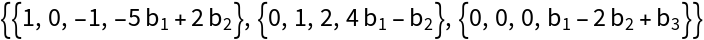When there are no constraint equations (i.e. when the system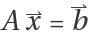is always consistent), the empty list is returned:

 In:=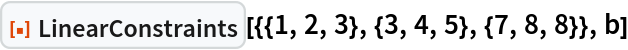Out=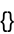### Applications (9)

#### Express the column space of a matrix as the null space of the coefficient matrix of its constraint equations (5)

The column space of a matrix is the null space of the coefficient matrix of its constraint equations. Begin with a matrix with rank 3:

 In:=Out=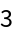The constraint equations are:

 In:=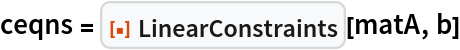Out=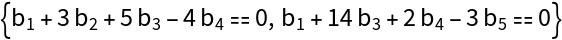The coefficient matrix of the constraint equations is:

 In:=Out=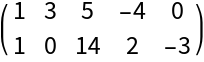The null space of this matrix is:

 In:=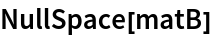Out=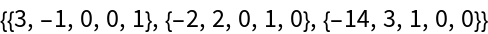These vectors are independent. To see that they are in the column space of the matrix A, simply verify that they satisfy the constraint equations:

 In:=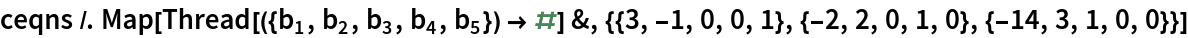Out=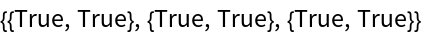#### Express the left null space of a matrix as the row space of the coefficient matrix of the constraint equations (4)

The left null space of a matrix is the row space of the coefficient matrix of its constraint equations. Begin with a matrix with rank 3:

 In:=Out=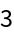The constraint equations are:

 In:=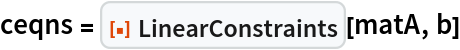Out=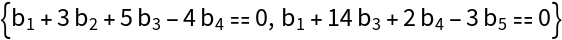The coefficient matrix of its constraint equations is:

 In:=Out=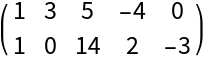The rows of this matrix span the left null space of A, since they are independent and satisfy BA=0:

 In:=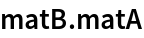Out=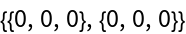## Publisher

Dennis M Schneider

## Version History

• 2.0.0 – 31 August 2020
• 1.0.0 – 28 August 2019